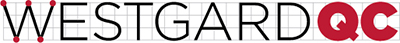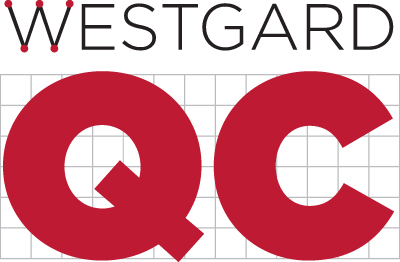QC Frequency Calculator
Analyst
Date
Analyzer
Test
Units
Levels of Controls Average
Concentration
Quality Requirement, TEa
Bias observed
Precision observed
Calculated Sigma-metric
Patient Risk Sigma
Applied Patient Risk Factor
Maximum Run Size
 Candidate SQC Procedures Run Size 1 Run Size 2 Run Size Av 1:3s/2:2s/R:4s/4:1s, N=4 (Pfr=0.03) 1:2.5s, N=4 (Pfr=0.04) 1:3s, N=4 (Pfr=0.01) 1:3s/2:2s/R:4s, N=2 (Pfr=0.01) 1:2.5s, N=2 (Pfr=0.02) 1:3s, N=2 (Pfr=0.00) 1:3.5s, N=2 (Pfr=0.00) 1:2s, N=1 (Pfr=0.05) 1:2.5s, N=1 (Pfr=0.01) 1:3s, N=1 (Pfr=0.00)